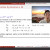Date
July 5 at 13:00 - 14:30, 2021 (JST)
Speaker
Dr. Myungbo Shim (Kyung Hee University, Republic of Korea)
Venue
via Zoom
Language
English

We propose a novel procedure of assigning a pair of non-unitary topological quantum field theories (TQFTs), TFT_\pm[T_0], to a (2+1)D interacting N=4 superconformal field theory (SCFT) T_0 of rank 0, i.e. having no Coulomb and Higgs branches. The topological theories arise from particular degenerate limits of the SCFT. Modular data of the non-unitary TQFTs are extracted from the supersymmetric partition functions in the degenerate limits. As a non-trivial dictionary, we propose that F = max{ -log |S^{(+)}_{0\alpha}| } = max{ -log |S^{(-)}_{0\alpha}| }, where F is the round three-sphere free energy of T_0 and S^{(\pm)}_{0\alpha} is the first column in the modular S-matrix of TFT_\pm. From the dictionary, we derive the lower bound on F, F > -log(\sqrt{(5-\sqrt{5})/10}) \simeq 0.642965, which holds for any rank 0 SCFT. The bound is saturated by the minimal N=4 SCFT proposed by Gang-Yamazaki, whose associated topological theories are both the Lee-Yang TQFT. We explicitly work out the (rank 0 SCFT)/(non-unitary TQFTs) correspondence for infinitely many examples. Before going to the technical part, we provide some background materials including some peculiar features in 3d gauge theories, some supersymmetries, anyons, and some modular data of MTC in this talk.

*If you would like to participate, please register using the form below.

## Reference